StatLect

# Trace of a matrix

The trace of a square matrix is the sum of its diagonal elements.

The trace enjoys several properties that are often very useful when proving results in matrix algebra and its applications.## Definition

Definition Letbe amatrix. Then, its trace, denoted byor, is the sum of its diagonal elements:## Examples

Some examples follow.

Example Define the matrixThen, its trace is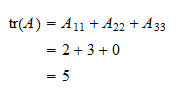Example Define the matrix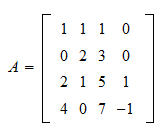Then, its trace is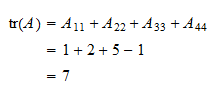## Properties

The following subsections report some useful properties of the trace operator.

### Trace of a sum

The trace of a sum of two matrices is equal to the sum of their trace.

Proposition Letandbe twomatrices. Then,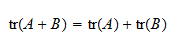Proof

Remember that the sum of two matrices is performed by summing each element of one matrix to the corresponding element of the other matrix (see the lecture on Matrix addition). As a consequence,### Trace of a scalar multiple

The next proposition tells us what happens to the trace when a matrix is multiplied by a scalar.

Proposition Letbe amatrix anda scalar. Then,Proof

Remember that the multiplication of a matrix by a scalar is performed by multiplying each entry of the matrix by the given scalar (see the lecture on Multiplication of a matrix by a scalar). As a consequence,### Trace of a linear combination

The two properties above (trace of sums and scalar multiples) imply that the trace of a linear combination is equal to the linear combination of the traces.

Proposition Letandbe twomatrices andandtwo scalars. Then,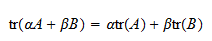### Trace of the transpose of a matrix

Transposing a matrix does not change its trace.

Proposition Letbe amatrix. Then,Proof

The trace of a matrix is the sum of its diagonal elements, but transposition leaves the diagonal elements unchanged.

### Trace of a product

The next proposition concerns the trace of a product of matrices.

Proposition Letbe amatrix andanmatrix. Then,Proof

Note thatis amatrix andis anmatrix. Then,where in stepsandwe have used the definition of matrix product, in particular, the facts thatis equal to the inner product between the-th row ofand the-th column of, andis equal to the inner product between the-th row ofand the-th column of.

### Trace of a scalar

A trivial, but often useful property is that a scalar is equal to its trace because a scalar can be thought of as amatrix, having a unique diagonal element, which in turn is equal to the trace.

This property is often used to write inner product as traces.

Example Letbe arow vector andacolumn vector. Then, the productis a scalar, and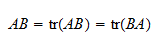where in the last step we have use the previous proposition on the product of traces. Thus, we have been able to write the scalaras the trace of thematrix.

## Solved exercises

Below you can find some exercises with explained solutions.

### Exercise 1

Letbe amatrix defined byFind its trace.

Solution

By summing the diagonal elements, we obtain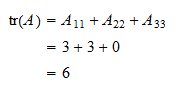### Exercise 2

Letbe amatrix andavector. Write the productas the trace of a product of twomatrices.

Solution

Sinceis a scalar, we have thatFurthermore,isandis. Therefore,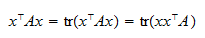where bothandare.

The book

Most of the learning materials found on this website are now available in a traditional textbook format.

Glossary entries
Share